# SAT December Score Releases, Upcoming Events!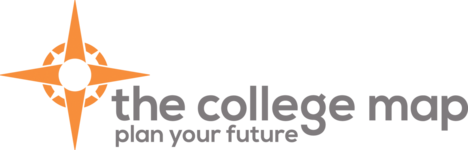/* styles */

# Hello, College Map!

 table div table+table+table+table div table{width:100%;padding:0}table div table+table+table+table div table img{width:96.23%;padding:0;float:none}table div table+table+table+table div table td{width:100%;padding:0 1.88% 18px}/* styles */## SAT SCORE REPORTINGDecember 1st SAT test day scores will be released on Friday, December 14th. Please take a moment to look up your scores in your collegeboard account and report them to your College Map consultant or update them in your CPP accounts under testing.

 table div table+table+table+table+table+table+table div table{width:100%;padding:0}table div table+table+table+table+table+table+table div table img{width:96.23%;padding:0;float:none}table div table+table+table+table+table+table+table div table td{width:100%;padding:0 1.88% 18px}/* styles */## December Scholarships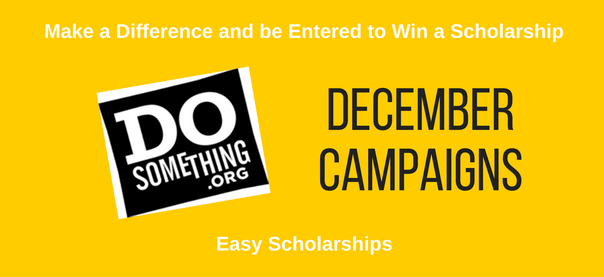Do you like making a difference in your school or community? If you do, DoSomething has many campaigns to make a difference, and for participating, you will be entered to win a scholarship! Below are campaigns from DoSomething that are accepting entries in December.

 table div table+table+table+table+table+table+table+table+table+table div table{width:100%;padding:0}table div table+table+table+table+table+table+table+table+table+table div table img{width:96.23%;padding:0;float:none}table div table+table+table+table+table+table+table+table+table+table div table td{width:100%;padding:0 1.88% 18px}/* styles */## UPCOMING DATES!The College Map will be closed beginning Friday, December 21, 2018 and will reopen January 7, 2019.

 table div table+table+table+table+table+table+table+table+table+table+table+table+table div table{width:100%;padding:0}table div table+table+table+table+table+table+table+table+table+table+table+table+table div table img{width:96.23%;padding:0;float:none}table div table+table+table+table+table+table+table+table+table+table+table+table+table div table td{width:100%;padding:0 1.88% 18px}/* styles */# Upcoming Events

Navigating High School Through the Eyes of a College Admissions Representative! This event is open to the community and families that are interested in learning more about the college admission process!
College Map Families, please share this event with anyone you know could benefit from this special event!

## Bright Futures Information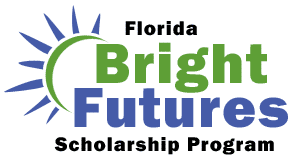/* styles */ Students must submit a completed Florida Financial Aid Application (FFAA) in order to be considered for State Scholarship & Grant Programs. Seniors, please Create a Student Account. After logging into your account, you may proceed to complete the FFAA. The Bright Futures link can answer all of your questions: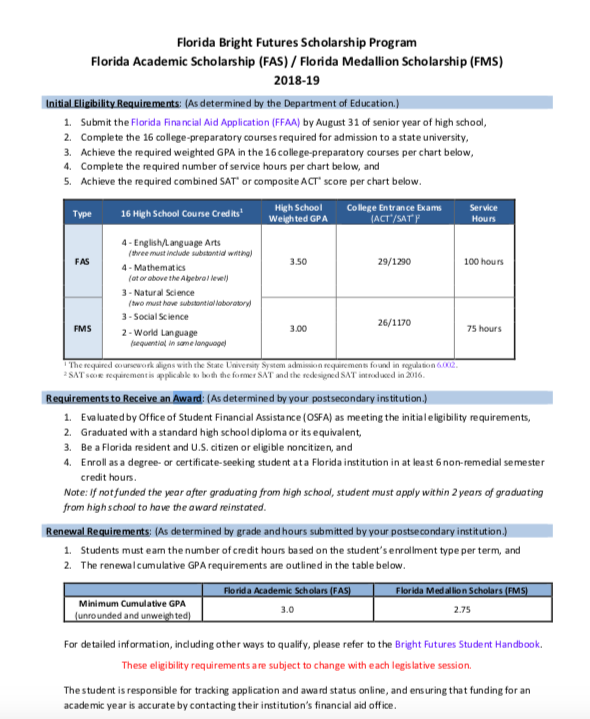table div table+table+table+table+table+table+table+table+table+table+table+table+table+table+table+table+table+table+table div table{width:100%;padding:0}table div table+table+table+table+table+table+table+table+table+table+table+table+table+table+table+table+table+table+table div table img{width:96.23%;padding:0;float:none}table div table+table+table+table+table+table+table+table+table+table+table+table+table+table+table+table+table+table+table div table td{width:100%;padding:0 1.88% 18px}/* styles */## JUNIORS/CLASS OF 2020 SUMMER DATES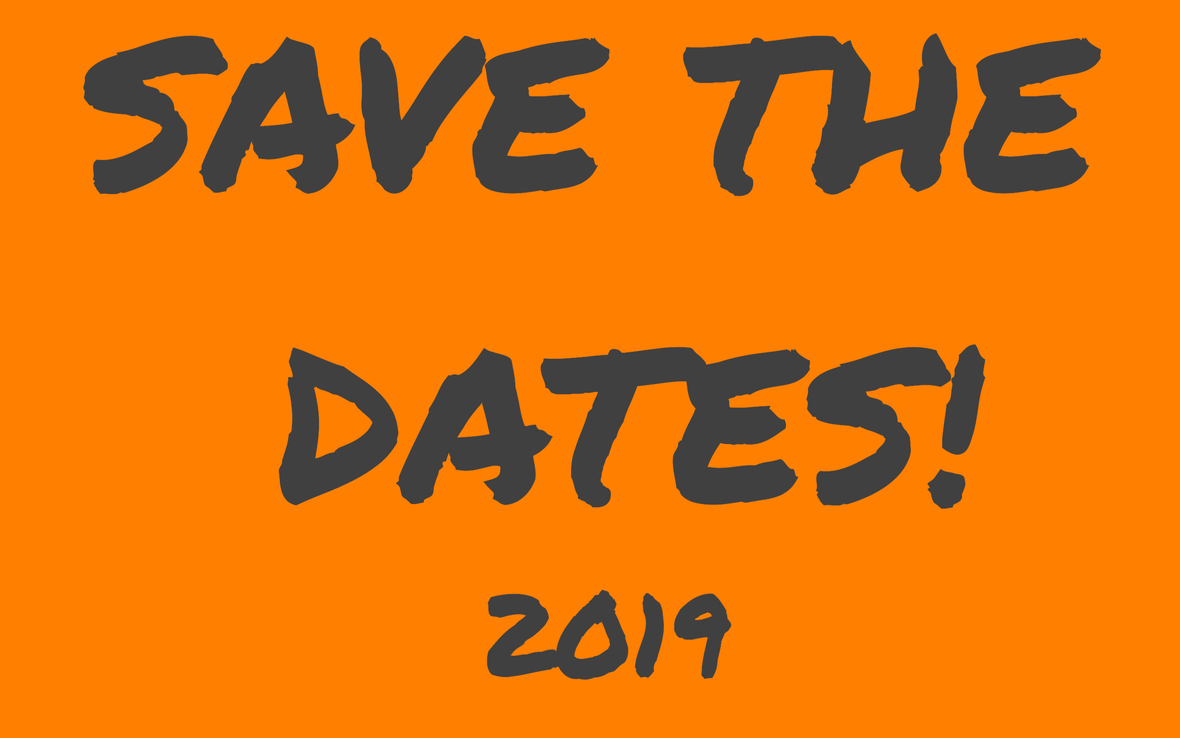/* styles */ ** JUNIORS/CLASS OF 2020 ESSAY WORKSHOP & SENIOR BOOT CAMPS DATES! Writing workshops will be held Saturday, May 18, 2019 and Saturday, June 1, 2019 from 10:00 a.m.until Noon. Students in the class of 2020 must attend one of these two workshops. Please mark your calendars and plan accordingly. Senior Boot Camps for the class of 2020 will be held for two weeks and we also ask that you plan your summer calendar with this in mind and attend all three days of at atleast one of the following weeks: July 23, 24 & 25 or July 30, 31 & August 1st. All dates will be 10:00 a.m. - 6:00 p.m.
 table div table+table+table+table+table+table+table+table+table+table+table+table+table+table+table+table+table+table+table+table+table+table+table div table{width:100%;padding:0}table div table+table+table+table+table+table+table+table+table+table+table+table+table+table+table+table+table+table+table+table+table+table+table div table img{width:96.23%;padding:0;float:none}table div table+table+table+table+table+table+table+table+table+table+table+table+table+table+table+table+table+table+table+table+table+table+table div table td{width:100%;padding:0 1.88% 18px}/* styles */## 2019 Test Prep Calendars

 /* styles */ Please sign up at our Acuity Scheduler website! See below for our new 2019 calendars. Please register for the ACT at act.org OR SAT at collegeboard.org. Please note there are early registration deadline dates. You can register after the deadline date but a late fee will be required.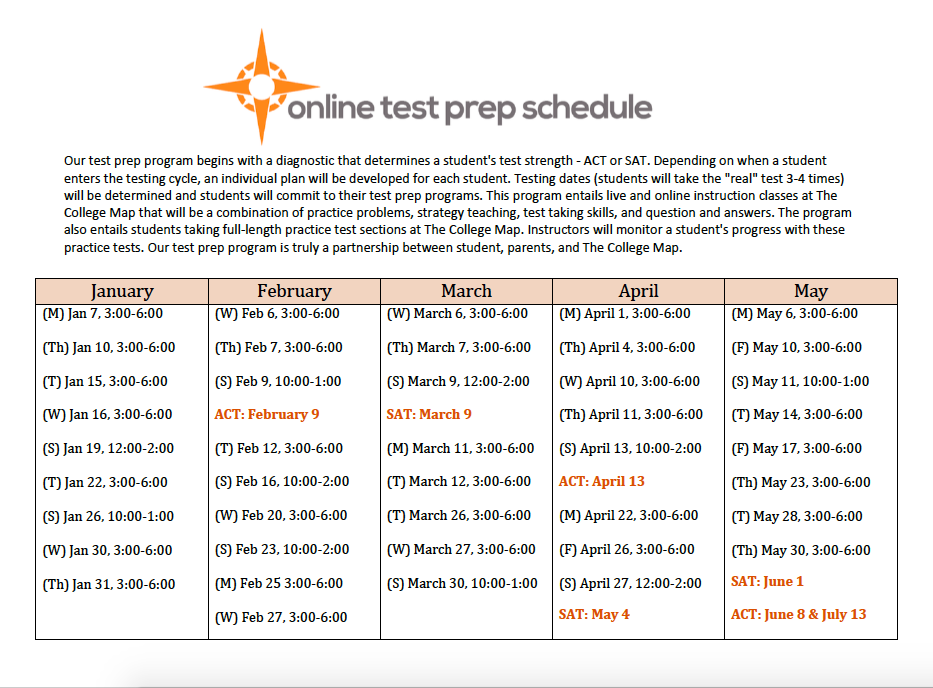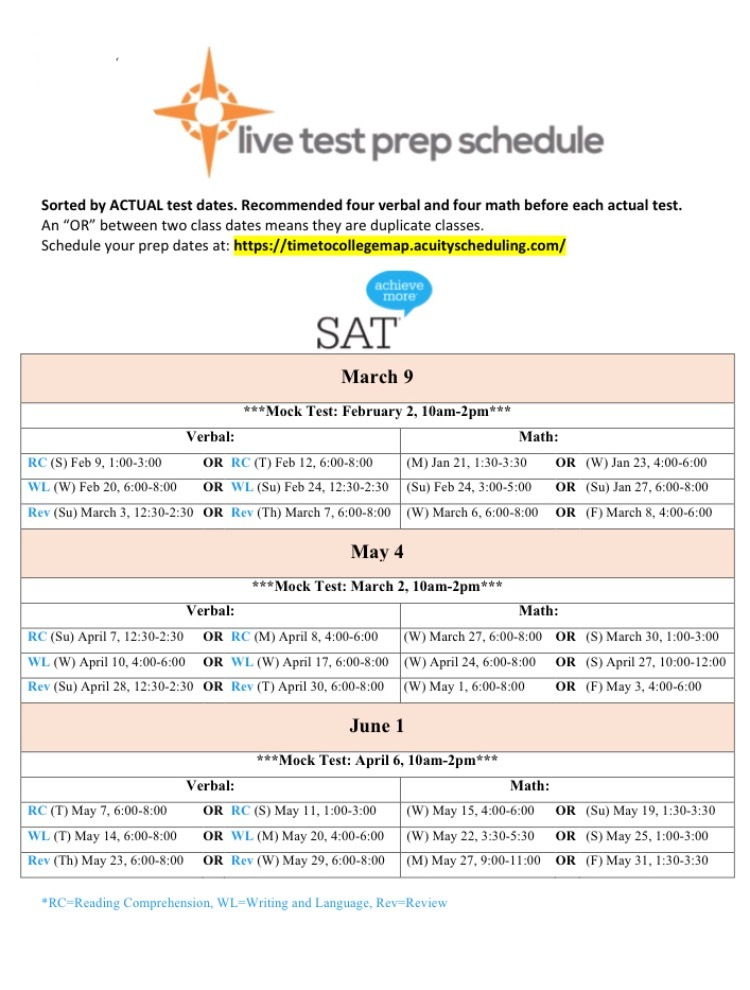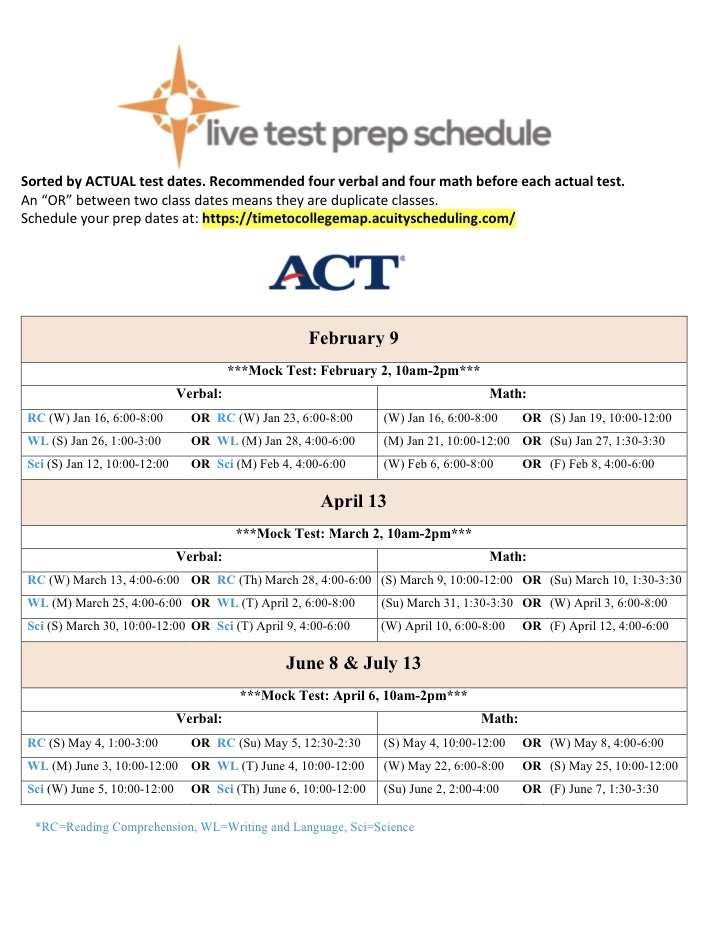table div table+table+table+table+table+table+table+table+table+table+table+table+table+table+table+table+table+table+table+table+table+table+table+table+table+table+table+table+table div table{width:100%;padding:0}table div table+table+table+table+table+table+table+table+table+table+table+table+table+table+table+table+table+table+table+table+table+table+table+table+table+table+table+table+table div table img{width:96.23%;padding:0;float:none}table div table+table+table+table+table+table+table+table+table+table+table+table+table+table+table+table+table+table+table+table+table+table+table+table+table+table+table+table+table div table td{width:100%;padding:0 1.88% 18px}/* styles */## JLV Scholarships!Great Resource for January 2019 Scholarships
Attending college can be quite expensive for students and their families. Luckily, there are many college scholarships and contests available to help pay for a college education. Students should seek out and apply for scholarships in which they meet the eligibility requirements. Click here for January 2019 due dates.

 table div table+table+table+table+table+table+table+table+table+table+table+table+table+table+table+table+table+table+table+table+table+table+table+table+table+table+table+table+table+table+table+table div table{width:100%;padding:0}table div table+table+table+table+table+table+table+table+table+table+table+table+table+table+table+table+table+table+table+table+table+table+table+table+table+table+table+table+table+table+table+table div table img{width:96.23%;padding:0;float:none}table div table+table+table+table+table+table+table+table+table+table+table+table+table+table+table+table+table+table+table+table+table+table+table+table+table+table+table+table+table+table+table+table div table td{width:100%;padding:0 1.88% 18px}/* styles */## Now What?Now What?

Seniors, as you wrap up your applications, you'll certainly feel like celebrating! But, this is no time to let down your guard. Colleges may communicate with you as they work through the admissions process. Pay close attention to:

Temporary student portal

It's a good idea to check these tools daily or at least several times a week! Click for more Additional Information!

 table div table+table+table+table+table+table+table+table+table+table+table+table+table+table+table+table+table+table+table+table+table+table+table+table+table+table+table+table+table+table+table+table+table+table+table div table{width:100%;padding:0}table div table+table+table+table+table+table+table+table+table+table+table+table+table+table+table+table+table+table+table+table+table+table+table+table+table+table+table+table+table+table+table+table+table+table+table div table img{width:96.23%;padding:0;float:none}table div table+table+table+table+table+table+table+table+table+table+table+table+table+table+table+table+table+table+table+table+table+table+table+table+table+table+table+table+table+table+table+table+table+table+table div table td{width:100%;padding:0 1.88% 18px}/* styles */## Scholarships Search Tools

 /* styles */ There are many good scholarship search tools/engines on the internet. Their primary function is to assist you in finding scholarships that match the eligibility criteria you enter for GPA, gender, residency, ethnicity/heritage, religion and area of study, hobbies, interests — some of the most common criteria used. The College Map team recommends the following search engines: Scholarships.com FastWeb Big Future Unigo SallieMae Please make sure to "click" or fill out as much information as possible. Once you've entered your information into the database, the search engine will generate a list of all current scholarships you qualify for. We recommend sorting that list by due date.
 table div table+table+table+table+table+table+table+table+table+table+table+table+table+table+table+table+table+table+table+table+table+table+table+table+table+table+table+table+table+table+table+table+table+table+table+table+table+table div table{width:100%;padding:0}table div table+table+table+table+table+table+table+table+table+table+table+table+table+table+table+table+table+table+table+table+table+table+table+table+table+table+table+table+table+table+table+table+table+table+table+table+table+table div table img{width:96.23%;padding:0;float:none}table div table+table+table+table+table+table+table+table+table+table+table+table+table+table+table+table+table+table+table+table+table+table+table+table+table+table+table+table+table+table+table+table+table+table+table+table+table+table div table td{width:100%;padding:0 1.88% 18px}/* styles */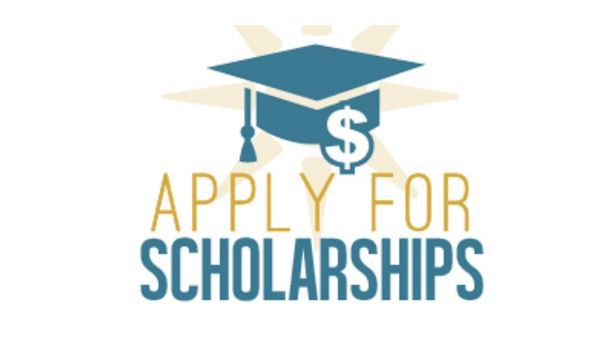# SENIORS! Institutional Scholarships

Institutional Scholarships are merit-based financial awards provided to you through the college/university you have applied to. Many of your schools will automatically consider you for scholarships, but please make sure that you are checking your individual school's websites for any additional freshman scholarships you may be eligible for that require a separate application. Several schools like, Auburn, Carnegie Mellon, Stetson and Alabama offer NON-automatic consideration scholarships.
We welcome seniors to attend boot camp on Wednesdays to work on scholarships after you have submitted your applications.

 table div table+table+table+table+table+table+table+table+table+table+table+table+table+table+table+table+table+table+table+table+table+table+table+table+table+table+table+table+table+table+table+table+table+table+table+table+table+table+table+table div table{width:100%;padding:0}table div table+table+table+table+table+table+table+table+table+table+table+table+table+table+table+table+table+table+table+table+table+table+table+table+table+table+table+table+table+table+table+table+table+table+table+table+table+table+table+table div table img{width:96.23%;padding:0;float:none}table div table+table+table+table+table+table+table+table+table+table+table+table+table+table+table+table+table+table+table+table+table+table+table+table+table+table+table+table+table+table+table+table+table+table+table+table+table+table+table+table div table td{width:100%;padding:0 1.88% 18px}/* styles */Our private "Your Path" page contains details for upcoming deadlines, events, and important announcements. We encourage you to check it regularly! The password is case-sensitive:
prepare@TCM2018

# Test Prep Schedules

Be sure to register for your spring test prep classes, available on the Your Path page and the Acuity Scheduler. Registration is mandatory; walk-ins may not be able to be accommodated.

# Suggestions?

Is there something you'd like to see in our newsletter? An event you'd like us to host? A friend you'd like to refer? Let us know by simply replying to this message.

 /* styles */ Kind regards, Your Team at The College Map